# GMAT Math : Calculating the slope of parallel lines

## Example Questions

### Example Question #1 : Calculating The Slope Of Parallel Lines

What is the slope of the line parallel to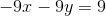?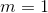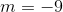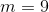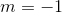Explanation:

Parallel lines have the same slope. Therefore, rewrite the equation in slope intercept form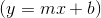: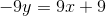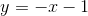### Example Question #2 : Calculating The Slope Of Parallel Lines

Find the slope of any line parallel to the following function.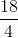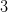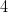Explanation:

We need to rearrange this equation to get intoform.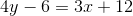Begin by adding 6 to both sides to get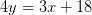Next, divide both sides by 4 to get our slope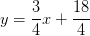So our slope, m, is equal to 3/4. Therefore, any line with the slope 3/4 will be parallel to the original function.

### Example Question #3 : Calculating The Slope Of Parallel Lines

A given line is defined by the equation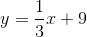. What is the slope of any line parallel to this line?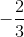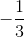Explanation:

Any line that is parallel to a linehas a slope that is equal to the slope. Given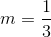and therefore any line parallel to the given line must have a slope of.

### Example Question #1 : Calculating The Slope Of Parallel Lines

A given line is defined by the equation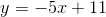. What is the slope of any line parallel to this line?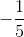Explanation:

Any line that is parallel to a linehas a slope that is equal to the slope. Given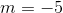and therefore any line parallel to the given line must have a slope of.

### Example Question #5 : Calculating The Slope Of Parallel Lines

A given line is defined by the equation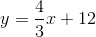. What is the slope of any line parallel to this line?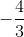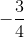Any line that is parallel to a linehas a slope that is equal to the slope. Given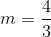and therefore any line parallel to the given line must have a slope of.